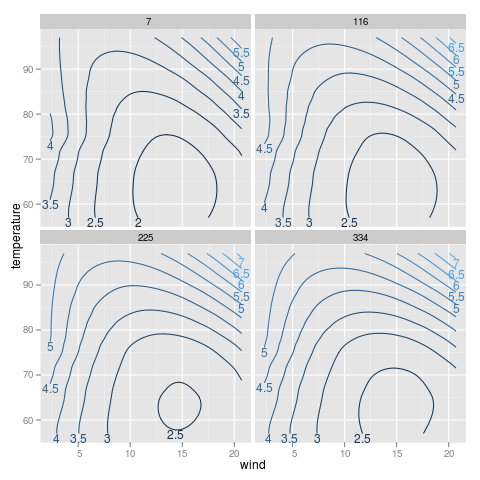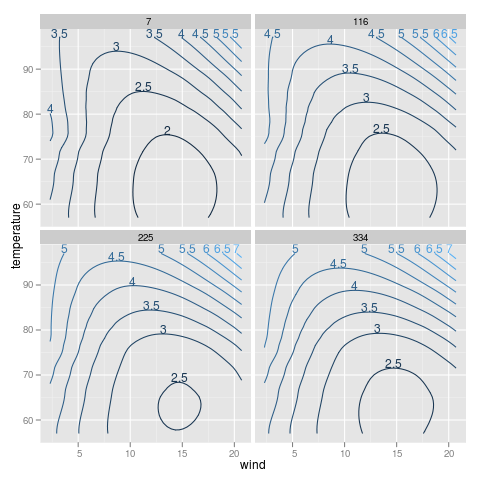# directlabels - contourplot - example - environmental

```## Example from help(contourplot)
require(stats)
require(lattice)
attach(environmental)
ozo.m <- loess((ozone^(1/3)) ~ wind * temperature * radiation,
parametric = c("radiation", "wind"), span = 1, degree = 2)
w.marginal <- seq(min(wind), max(wind), length.out = 50)
t.marginal <- seq(min(temperature), max(temperature), length.out = 50)Positioning Method for the bottom of a group of points. ```direct.label(p,"bottom.pieces") ```Positioning Method for the top of a group of points. ```direct.label(p,"top.pieces") ```